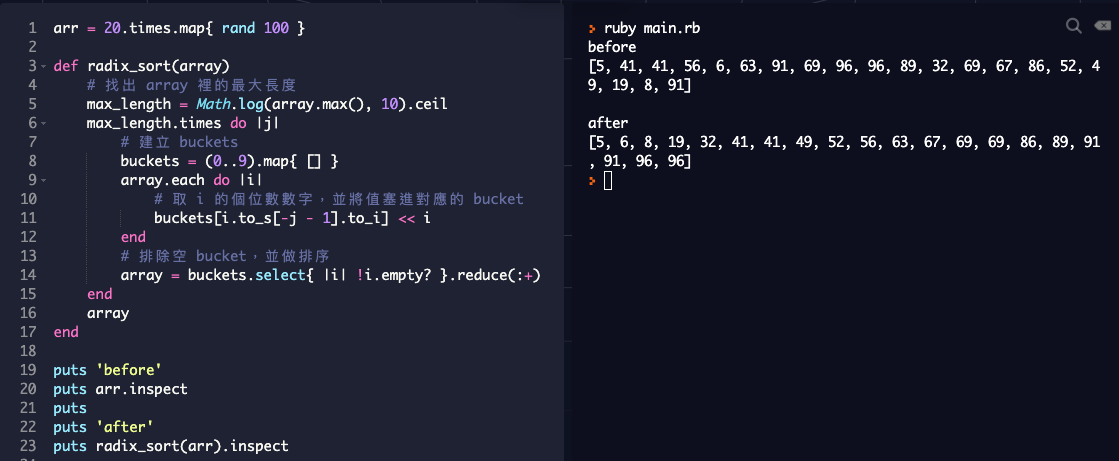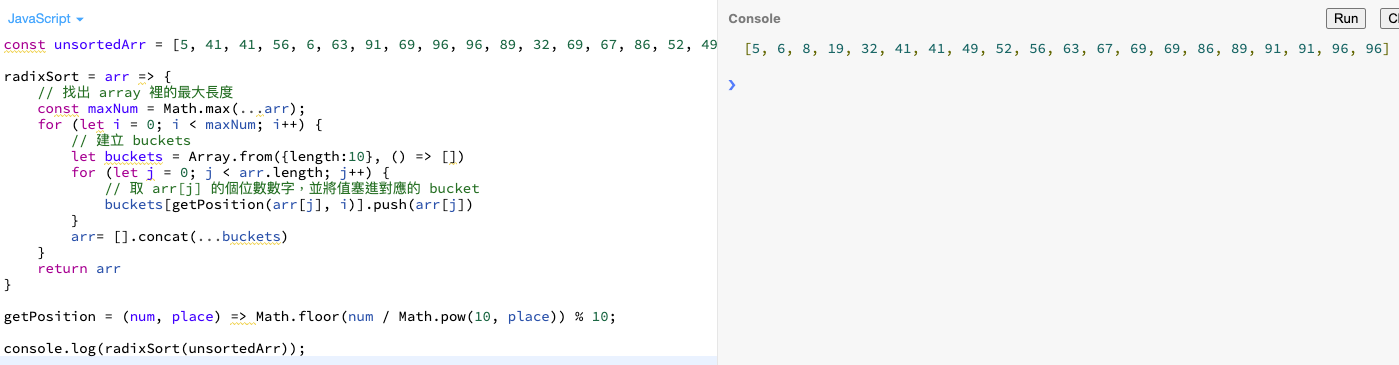# Stan Blog

## 學習過程中的一些記錄

Radix Sort 的定義 (參考維基百科)

``````原理是將整數按位元數切割成不同的數字，然後按每個位數分別比較。

``````

1. 取得每個資料鍵值的最 小/大 位數

2. 依據該位數大小排序資料

3. 取得下一個有效位數，並重複步驟二，至最 小/大 位數為止#### 範例程式碼

##### Ruby 範例

``````arr = 20.times.map{ rand 100 }

def radix_sort(array)
# 找出 array 裡的最大長度
max_length = Math.log(array.max(), 10).ceil
max_length.times do |j|
# 建立 buckets
buckets = (0..9).map{ [] }
array.each do |i|
# 取 i 的個位數數字，並將值塞進對應的 bucket
buckets[i.to_s[-j - 1].to_i] << i
end
# 排除空 bucket，並做排序
array = buckets.select{ |i| !i.empty? }.reduce(:+)
end
array
end

puts 'before'
puts arr.inspect
puts
puts 'after'
puts radix_sort(arr).inspect
``````##### JavaScript 範例

JavaScript 的 online sandbox，有興趣可以把 code 貼進去玩玩看

``````const unsortedArr = [5, 41, 41, 56, 6, 63, 91, 69, 96, 96, 89, 32, 69, 67, 86, 52, 49, 19, 8, 91];

radixSort = arr => {
// 找出 array 裡的最大長度
const maxNum = Math.max(...arr);
for (let i = 0; i < maxNum; i++) {
// 建立 buckets
let buckets = Array.from({length:10}, () => [])
for (let j = 0; j < arr.length; j++) {
// 取 arr[j] 的個位數數字，並將值塞進對應的 bucket
buckets[getPosition(arr[j], i)].push(arr[j])
}
arr= [].concat(...buckets)
}
return arr
}

getPosition = (num, place) => Math.floor(num / Math.pow(10, place)) % 10;

console.log(radixSort(unsortedArr));
``````#### Comments

comments powered by Disqus